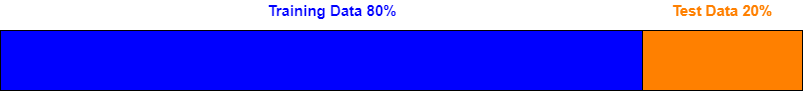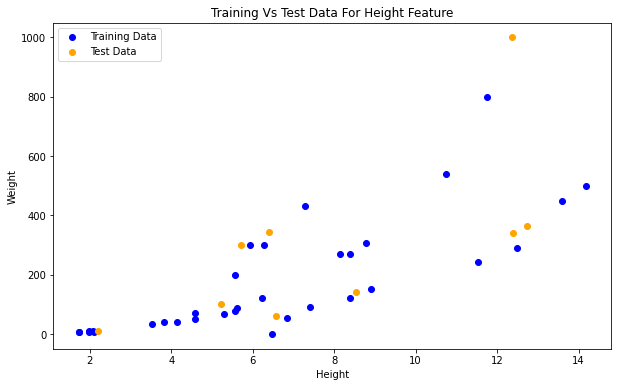# Train Test SplitIn machine learning we build model based on given data, but to test the performance of the model we also need test data. Technically we can use the same data for model performance testing but the results won’t be reliable. Recommended way is to use the different set of data for model training and model performance testing. Datasets used for model training are called as ‘Training Datasets’ and datasets used for testing are called as ‘Test Datasets’

## Train and Test Datasets

We usually do 80-20 split for training and test datasets. Its is also good practice to randomly sort the data before splitting into two datasets. We are going to use Sklearn library (model_selection.train_test_split) for splitting the datasets.## Python Code

### Import Libraries

• pandas: Used for data manipulation and analysis.
• train_test_split: Sklearn train_test_split is used to split the dataset
• linear_model: Sklearn linear regression model
``````import pandas as pd
from sklearn.model_selection import train_test_split
from sklearn import linear_model
``````

### Import Dataset

``````df = pd.read_csv('https://raw.githubusercontent.com/satishgunjal/datasets/master/Fish_Weight_Train_Test_Split.csv')
print('Dimension of dataset= ', df.shape)
df.head(5) # Show first 5 training examples
``````
``````Dimension of dataset=  (42, 3)
``````
Weight Height Width
0 242.0 11.5200 4.0200
1 290.0 12.4800 4.3056
2 340.0 12.3778 4.6961
3 363.0 12.7300 4.4555
4 450.0 13.6024 4.9274

### Understanding The Dataset

• There are total 42 rows(training samples) and 4 columns in dataset.
• Features/input values/independent variables are ‘Height’ and ‘Width’
• Labels/Target/output value/dependent variable is ‘Weight’

Let’s create separate dataframe for features and labels. It is required for splitting the dataset.

``````X = df.drop(['Weight'], axis='columns')
X.head(5)
``````
Height Width
0 11.5200 4.0200
1 12.4800 4.3056
2 12.3778 4.6961
3 12.7300 4.4555
4 13.6024 4.9274
``````y = df.Weight
y.head()
``````
``````0    242.0
1    290.0
2    340.0
3    363.0
4    450.0
Name: Weight, dtype: float64
``````

Now we have features and target variables ready, lets split the data into training and test datasets

### Using Sklearn train_test_split Method

• train_test_split() method takes three arguments input features, labels and test_size.
• Test size determines the percentage of split. e.g. test_size = 0.2, means 80% training data and 20% test data.
• random_state is optional argument.

### What Is random_state

• It is used for initializing the internal random number generator, which will decide the splitting of data into train and test datasets
• Order of the data will be same for a particular value of random_state. For e.g. for ‘random_state=1’ no matter how many times you run the code you will get same data in training and test split
• You can use any integer value for random_state. Just remember one thing if you don’t pass any value, then it will use default value ‘None’ and split data randomly every time you execute the code.
``````X_train, X_test, y_train, y_test = train_test_split(X, y, test_size = 0.2, random_state = 1)

print('X_train dimension= ', X_train.shape)
print('X_test dimension= ', X_test.shape)
print('y_train dimension= ', y_train.shape)
print('y_train dimension= ', y_test.shape)
``````
``````X_train dimension=  (33, 2)
X_test dimension=  (9, 2)
y_train dimension=  (33,)
y_train dimension=  (9,)
``````

Lets visulaize the training and test data using scatter plot

``````import matplotlib.pyplot as plt
plt.scatter(X_train.Height,y_train, color='blue', label='Training Data')
plt.scatter(X_test.Height,y_test, color='orange', label='Test Data')
plt.xlabel('Height')
plt.ylabel('Weight')
plt.title('Training Vs Test Data For Height Feature')
plt.rcParams["figure.figsize"] = (10,6)
plt.legend()
``````### Linear Model Training Using Training Dataset

Since we have training and test dataset ready, lets use training dataset for linear model training.

``````lm = linear_model.LinearRegression()
lm.fit(X_train, y_train)
``````
``````LinearRegression(copy_X=True, fit_intercept=True, n_jobs=None, normalize=False)
``````

### Linear Model Testing Using Test Dataset

Lets use test dataset for linear model performance testing.

``````lm.score(X_test, y_test)
``````
``````0.7153810385773975
``````

### Linear Model Testing Using Training Dataset

Lets use training dataset for linear model performance testing. Notice the difference in performance score.

``````lm.score(X_train, y_train)
``````
``````0.8488919680474343
``````

### Never Test On Training Data

• As you can notice score with training data is higher than score with test data.
• Higher score is misleading in this case.
• Model which dont use separate dataset for testing may have higher performance score but it wont generalize well and give misleading predictions with real world data.

Hence forward, in all the tutorials we are going to use training and test dataset for model training and testing.

## Learning Path for DP-900 Microsoft Azure Data Fundamentals Certification

8 minute read

Learning path to gain necessary skills and to clear the Azure Data Fundamentals Certification. This certification is intended for candidates beginning to wor...

## Learning Path for AI-900 Microsoft Azure AI Fundamentals Certification

7 minute read

Learning path to gain necessary skills and to clear the Azure AI Fundamentals Certification. This certification is intended for candidates with both technica...

## ANN Model to Classify Images

12 minute read

In this guide we are going to create and train the neural network model to classify the clothing images. We will use TensorFlow deep learning framework along...

## Introduction to NLP

8 minute read

In short NLP is an AI technique used to do text analysis. Whenever we have lots of text data to analyze we can use NLP. Apart from text analysis, NLP also us...

## K Fold Cross Validation

14 minute read

There are multiple ways to split the data for model training and testing, in this article we are going to cover K Fold and Stratified K Fold cross validation...

## K-Means Clustering

13 minute read

K-Means clustering is most commonly used unsupervised learning algorithm to find groups in unlabeled data. Here K represents the number of groups or clusters...

## Time Series Analysis and Forecasting

10 minute read

Any data recorded with some fixed interval of time is called as time series data. This fixed interval can be hourly, daily, monthly or yearly. Objective of t...

## Support Vector Machines

9 minute read

Support vector machines is one of the most powerful ‘Black Box’ machine learning algorithm. It belongs to the family of supervised learning algorithm. Used t...

## Random Forest

12 minute read

Random forest is supervised learning algorithm and can be used to solve classification and regression problems. Unlike decision tree random forest fits multi...

## Decision Tree

13 minute read

Decision tree explained using classification and regression example. The objective of decision tree is to split the data in such a way that at the end we hav...

## Agile Scrum Framework

7 minute read

This tutorial covers basic Agile principles and use of Scrum framework in software development projects.

## Underfitting & Overfitting

2 minute read

Main objective of any machine learning model is to generalize the learning based on training data, so that it will be able to do predictions accurately on un...

## Multiclass Logistic Regression Using Sklearn

6 minute read

In this study we are going to use the Linear Model from Sklearn library to perform Multi class Logistic Regression. We are going to use handwritten digit’s d...

## Binary Logistic Regression Using Sklearn

6 minute read

In this tutorial we are going to use the Logistic Model from Sklearn library. We are also going to use the same test data used in Logistic Regression From Sc...

## Logistic Regression From Scratch With Python

14 minute read

This tutorial covers basic concepts of logistic regression. I will explain the process of creating a model right from hypothesis function to algorithm. We wi...

## Train Test Split

3 minute read

In this tutorial we are going to study about train, test data split. We will use sklearn library to do the data split.

## One Hot Encoding

11 minute read

In this tutorial we are going to study about One Hot Encoding. We will also use pandas and sklearn libraries to convert categorical data into numeric data.

## Multivariate Linear Regression Using Scikit Learn

8 minute read

In this tutorial we are going to use the Linear Models from Sklearn library. Scikit-learn is one of the most popular open source machine learning library for...

## Univariate Linear Regression Using Scikit Learn

7 minute read

In this tutorial we are going to use the Linear Models from Sklearn library. Scikit-learn is one of the most popular open source machine learning library for...

## Multivariate Linear Regression From Scratch With Python

10 minute read

In this tutorial we are going to cover linear regression with multiple input variables. We are going to use same model that we have created in Univariate Lin...

## Univariate Linear Regression From Scratch With Python

13 minute read

This tutorial covers basic concepts of linear regression. I will explain the process of creating a model right from hypothesis function to gradient descent a...

## Machine Learning Introduction And Learning Plan

4 minute read

In this tutorial we will see the brief introduction of Machine Learning and preferred learning plan for beginners

Back to top ↑